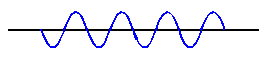# sine wave

(redirected from Sine-wave)
Also found in: Dictionary, Thesaurus, Medical, Financial.
Related to Sine-wave: Sinusoidal wave

## Sine wave

A wave having a form which, if plotted, would be the same as that of a trigonometric sine or cosine function. The sine wave may be thought of as the projection on a plane of the path of a point moving around a circle at uniform speed. It is characteristic of one-dimensional vibrations and one-dimensional waves having no dissipation. See Harmonic motion

The sine wave is the basic function employed in harmonic analysis. It can be shown that any complex motion in a one-dimensional system can be described as the superposition of sine waves having certain amplitude and phase relationships. The technique for determining these relationships is known as Fourier analysis. See Wave equation, Wave motion

## sine wave

[′sīn ‚wāv]
(physics)
A wave whose amplitude varies as the sine of a linear function of time. Also known as sinusoidal wave.

## sine wave

A wave form containing only one frequency; the amplitude of the periodic oscillation is a sinusoidal function of time. Also see pure tone.

## sine wave

(mathematics)
A waveform of a single constant frequency and amplitude that continues for all time.

Compare wavelet.

## sine wave

A continuous, uniform wave with a constant frequency and amplitude. See wavelength.

A Sine WaveReferences in periodicals archive ?
5 hp well pump- with a Xantrex SW 4024 sine-wave inverter (offering 4,000 watts of continuous output) and a 120/240volt transformer.
This paper analyzes empirical constraints from the shape of the contrast sensitivity function (CSF) at several illuminance levels and from the relationship between illuminance and visual acuity, showing that the DeVries-Rose to Weber transition with sine-wave gratings can only hold at very low spatial frequencies.
We returned the modified sine-wave inverter to the dealer and replaced it with a pure sine-wave device, which was twice as expensive but well worth the cost.
In the NI demo, a Compact RIO system induces a 200-kHz sine wave on the saw blade through a known impedance and monitors the sine-wave amplitude at the blade.
Absopulse Electronics' CSI 2K-6KM Series sine-wave output inverter system was designed for operation in industrial, oil and gas, mining, mobile, utility, telecom and other heavyduty environments.

Site: Follow: Share:
Open / Close